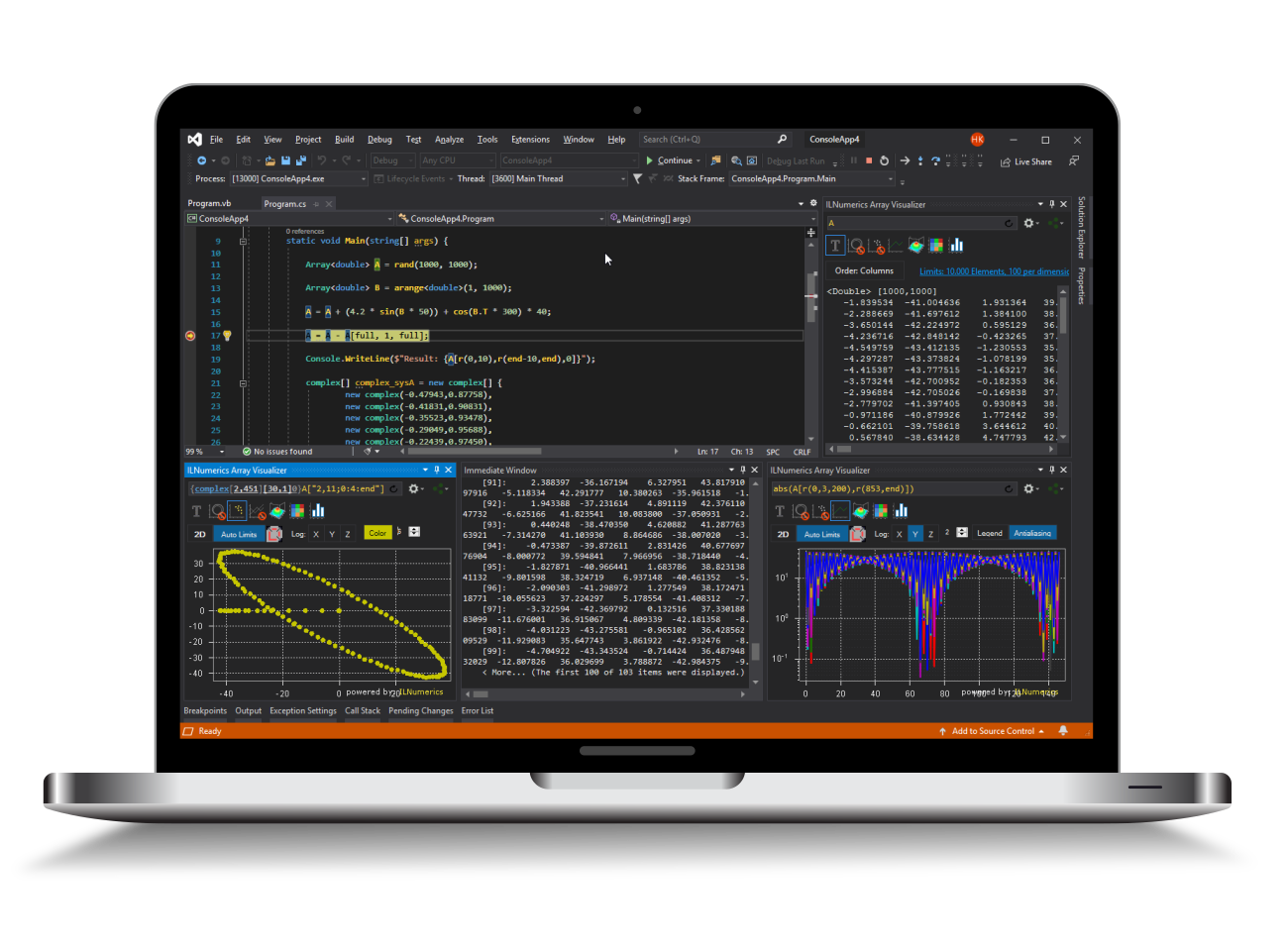Industrial Data Science
in C# and .NET:
Simple. Fast. Reliable.ILNumerics - Technical Computing

Modern High Performance Tools for Technical

Computing and Visualization in Industry and Science

# Nonlinear Equation System PDL Least Squares Solver

ILNumerics Optimization Toolbox is used to solve a system of nonlinear equations F(x) = 0. The equations are wrapped into a dedicated class and assemble a cost function which returns the output of 4 nonlinear functions according to the input of a 4 element vector. The Powell's Dog Leg least squares solver is used to find the minimum of

argmin { || F(x) ||^2, subject to x in R^n

The minimizer found corresponds to the solution x of the original equation F(x) = 0. The example is easily extensible to more dimensions and arbitrary cost functions F(x).